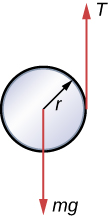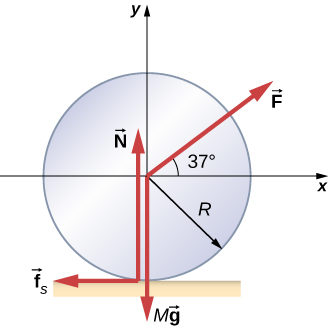# 11.1 Rolling motion  (Page 5/6)

 Page 5 / 6
${v}_{\text{CM}}=\sqrt{\left(3.71\phantom{\rule{0.2em}{0ex}}\text{m}\text{/}{\text{s}}^{2}\right)25.0\phantom{\rule{0.2em}{0ex}}\text{m}}=9.63\phantom{\rule{0.2em}{0ex}}\text{m}\text{/}\text{s}\text{.}$

## Significance

This is a fairly accurate result considering that Mars has very little atmosphere, and the loss of energy due to air resistance would be minimal. The result also assumes that the terrain is smooth, such that the wheel wouldn’t encounter rocks and bumps along the way.

Also, in this example, the kinetic energy, or energy of motion, is equally shared between linear and rotational motion. If we look at the moments of inertia in [link] , we see that the hollow cylinder has the largest moment of inertia for a given radius and mass. If the wheels of the rover were solid and approximated by solid cylinders, for example, there would be more kinetic energy in linear motion than in rotational motion. This would give the wheel a larger linear velocity than the hollow cylinder approximation. Thus, the solid cylinder would reach the bottom of the basin faster than the hollow cylinder.

## Summary

• In rolling motion without slipping, a static friction force is present between the rolling object and the surface. The relations ${v}_{\text{CM}}=R\omega ,{a}_{\text{CM}}=R\alpha ,\phantom{\rule{0.2em}{0ex}}\text{and}\phantom{\rule{0.2em}{0ex}}{d}_{\text{CM}}=R\theta$ all apply, such that the linear velocity, acceleration, and distance of the center of mass are the angular variables multiplied by the radius of the object.
• In rolling motion with slipping, a kinetic friction force arises between the rolling object and the surface. In this case, ${v}_{\text{CM}}\ne R\omega ,{a}_{\text{CM}}\ne R\alpha ,\phantom{\rule{0.2em}{0ex}}\text{and}\phantom{\rule{0.2em}{0ex}}{d}_{\text{CM}}\ne R\theta$ .
• Energy conservation can be used to analyze rolling motion. Energy is conserved in rolling motion without slipping. Energy is not conserved in rolling motion with slipping due to the heat generated by kinetic friction.

## Conceptual questions

Can a round object released from rest at the top of a frictionless incline undergo rolling motion?

No, the static friction force is zero.

A cylindrical can of radius R is rolling across a horizontal surface without slipping. (a) After one complete revolution of the can, what is the distance that its center of mass has moved? (b) Would this distance be greater or smaller if slipping occurred?

A wheel is released from the top on an incline. Is the wheel most likely to slip if the incline is steep or gently sloped?

The wheel is more likely to slip on a steep incline since the coefficient of static friction must increase with the angle to keep rolling motion without slipping.

Which rolls down an inclined plane faster, a hollow cylinder or a solid sphere? Both have the same mass and radius.

A hollow sphere and a hollow cylinder of the same radius and mass roll up an incline without slipping and have the same initial center of mass velocity. Which object reaches a greater height before stopping?

The cylinder reaches a greater height. By [link] , its acceleration in the direction down the incline would be less.

## Problems

What is the angular velocity of a 75.0-cm-diameter tire on an automobile traveling at 90.0 km/h?

${v}_{\text{CM}}=R\omega \phantom{\rule{0.2em}{0ex}}⇒\omega =66.7\phantom{\rule{0.2em}{0ex}}\text{rad/s}$

A boy rides his bicycle 2.00 km. The wheels have radius 30.0 cm. What is the total angle the tires rotate through during his trip?

If the boy on the bicycle in the preceding problem accelerates from rest to a speed of 10.0 m/s in 10.0 s, what is the angular acceleration of the tires?

$\alpha =3.3\phantom{\rule{0.2em}{0ex}}\text{rad}\text{/}{\text{s}}^{2}$

Formula One race cars have 66-cm-diameter tires. If a Formula One averages a speed of 300 km/h during a race, what is the angular displacement in revolutions of the wheels if the race car maintains this speed for 1.5 hours?

A marble rolls down an incline at $30\text{°}$ from rest. (a) What is its acceleration? (b) How far does it go in 3.0 s?

${I}_{\text{CM}}=\frac{2}{5}m{r}^{2},\phantom{\rule{0.2em}{0ex}}{a}_{\text{CM}}=3.5\phantom{\rule{0.2em}{0ex}}\text{m}\text{/}{\text{s}}^{2};\phantom{\rule{0.2em}{0ex}}x=15.75\phantom{\rule{0.2em}{0ex}}\text{m}$

Repeat the preceding problem replacing the marble with a solid cylinder. Explain the new result.

A rigid body with a cylindrical cross-section is released from the top of a $30\text{°}$ incline. It rolls 10.0 m to the bottom in 2.60 s. Find the moment of inertia of the body in terms of its mass m and radius r.

positive is down the incline plane;
${a}_{\text{CM}}=\frac{mg\phantom{\rule{0.2em}{0ex}}\text{sin}\phantom{\rule{0.2em}{0ex}}\theta }{m+\left({I}_{\text{CM}}\text{/}{r}^{2}\right)}⇒{I}_{\text{CM}}={r}^{2}\left[\frac{mg\phantom{\rule{0.2em}{0ex}}\text{sin}30}{{a}_{\text{CM}}}-m\right]$ ,
$x-{x}_{0}={v}_{0}t-\frac{1}{2}{a}_{\text{CM}}{t}^{2}⇒{a}_{\text{CM}}=2.96\phantom{\rule{0.2em}{0ex}}{\text{m/s}}^{2},$
${I}_{\text{CM}}=0.66\phantom{\rule{0.2em}{0ex}}m{r}^{2}$

A yo-yo can be thought of a solid cylinder of mass m and radius r that has a light string wrapped around its circumference (see below). One end of the string is held fixed in space. If the cylinder falls as the string unwinds without slipping, what is the acceleration of the cylinder?A solid cylinder of radius 10.0 cm rolls down an incline with slipping. The angle of the incline is $30\text{°}.$ The coefficient of kinetic friction on the surface is 0.400. What is the angular acceleration of the solid cylinder? What is the linear acceleration?

$\alpha =67.9\phantom{\rule{0.2em}{0ex}}\text{rad}\text{/}{\text{s}}^{2}$ ,
${\left({a}_{\text{CM}}\right)}_{x}=1.5\phantom{\rule{0.2em}{0ex}}\text{m}\text{/}{\text{s}}^{2}$

A bowling ball rolls up a ramp 0.5 m high without slipping to storage. It has an initial velocity of its center of mass of 3.0 m/s. (a) What is its velocity at the top of the ramp? (b) If the ramp is 1 m high does it make it to the top?

A 40.0-kg solid cylinder is rolling across a horizontal surface at a speed of 6.0 m/s. How much work is required to stop it?

$W=-1080.0\phantom{\rule{0.2em}{0ex}}\text{J}$

A 40.0-kg solid sphere is rolling across a horizontal surface with a speed of 6.0 m/s. How much work is required to stop it? Compare results with the preceding problem.

A solid cylinder rolls up an incline at an angle of $20\text{°}.$ If it starts at the bottom with a speed of 10 m/s, how far up the incline does it travel?

Mechanical energy at the bottom equals mechanical energy at the top;
$\frac{1}{2}m{v}_{0}^{2}+\frac{1}{2}\left(\frac{1}{2}m{r}^{2}\right){\left(\frac{{v}_{0}}{r}\right)}^{2}=mgh⇒h=\frac{1}{g}\left(\frac{1}{2}+\frac{1}{4}\right){v}_{0}^{2}$ ,
$h=7.7\phantom{\rule{0.2em}{0ex}}\text{m,}$ so the distance up the incline is $22.5\phantom{\rule{0.2em}{0ex}}\text{m}$ .

A solid cylindrical wheel of mass M and radius R is pulled by a force $\stackrel{\to }{F}$ applied to the center of the wheel at $37\text{°}$ to the horizontal (see the following figure). If the wheel is to roll without slipping, what is the maximum value of $|\stackrel{\to }{F}|?$ The coefficients of static and kinetic friction are ${\mu }_{\text{S}}=0.40\phantom{\rule{0.2em}{0ex}}\text{and}\phantom{\rule{0.2em}{0ex}}{\mu }_{\text{k}}=0.30.$A hollow cylinder is given a velocity of 5.0 m/s and rolls up an incline to a height of 1.0 m. If a hollow sphere of the same mass and radius is given the same initial velocity, how high does it roll up the incline?

Use energy conservation
$\frac{1}{2}m{v}_{0}^{2}+\frac{1}{2}{I}_{\text{Cyl}}{\omega }_{0}^{2}=mg{h}_{\text{Cyl}}$ ,
$\frac{1}{2}m{v}_{0}^{2}+\frac{1}{2}{I}_{\text{Sph}}{\omega }_{0}^{2}=mg{h}_{\text{Sph}}$ .
Subtracting the two equations, eliminating the initial translational energy, we have
$\frac{1}{2}{I}_{\text{Cyl}}{\omega }_{0}^{2}-\frac{1}{2}{I}_{\text{Sph}}{\omega }_{0}^{2}=mg\left({h}_{\text{Cyl}}-{h}_{\text{Sph}}\right)$ ,
$\frac{1}{2}m{r}^{2}{\left(\frac{{v}_{0}}{r}\right)}^{2}-\frac{1}{2}\frac{2}{3}m{r}^{2}{\left(\frac{{v}_{0}}{r}\right)}^{2}=mg\left({h}_{\text{Cyl}}-{h}_{\text{Sph}}\right)$ ,
$\frac{1}{2}{v}_{0}^{2}-\frac{1}{2}\frac{2}{3}{v}_{0}^{2}=g\left({h}_{\text{Cyl}}-{h}_{\text{Sph}}\right)$ ,
${h}_{\text{Cyl}}-{h}_{\text{Sph}}=\frac{1}{g}\left(\frac{1}{2}-\frac{1}{3}\right){v}_{0}^{2}=\frac{1}{9.8\phantom{\rule{0.2em}{0ex}}\text{m}\text{/}{\text{s}}^{2}}\left(\frac{1}{6}\right)\left(5.0\phantom{\rule{0.2em}{0ex}}\text{m}\text{/}{\text{s)}}^{2}=0.43\phantom{\rule{0.2em}{0ex}}\text{m}$ .
Thus, the hollow sphere, with the smaller moment of inertia, rolls up to a lower height of $1.0-0.43=0.57\phantom{\rule{0.2em}{0ex}}\text{m}\text{.}$

what is mass
it is the quantity of matter in a substance....expressed in Kg as the SI unit.
Carloes
what is the universe of conductivity
what is si unit of force?
Kg m / s^2 You can see that this is the case from dimensional analysis of F = M a
Peter
Newton which is Kgm/s2
NAWED
newton(N)
Carloes
What's power in physics
Richard
newton(N)
Shahzab
Power is the rate of doing work. P=W/T
DOLLY
A car moving with a velocity of 54km/hr accelerates uniformly at the rate of 2mper seconds. Calculate the distance traveled from the place where acceleration began to where the velocity reaches 72km/hr and the time taken to cover d distance
t=2.5s and s=43.75m
Ajayi
how to find amplitude of interfering wave
how do we slove a pendulum clock shaft question
An object of mass m moves with acceleration a down a rough incline.
If the average velocity of an object is zero in some time interval, what can you say about the displacement of the object fr that interval?
Phyu
what is force
you ever seen star wars?
Jake
Yes
okon
What is angular velocity
refer to how fast an object rotates ot revolve to another point
samson
An object undergoes constant acceleration after starting from rest and then travels 5m in the first seconds .determine how far it will go in the next seconds
x=x0+v0t+1/2at^2
Grant
what is the deference between precision and accuracy
ShAmy
In measurement of a set, accuracy refers to closeness of the measurements to a specific value, while precision refers to the closeness of the measurements to each other.
Grant
Thank you Mr..
ShAmy
Are there is a difference between Error and Relative Error...
ShAmy
An aircraft flies 300km due east and 600km due north. determine the magnitude of its displacement
670.8km
iyiola
which formula did you use
Sophy
X^2=300^2+600^2
iyiola
what is Fermat principle.
find the angle of projection at which the horizontal range is twice the maximum height of a projectile
impulse by height fomula
Hello
what is impulse?
impulse is the integral of a force (F),over the interval for which it act
AgbeyangiByByBy Dravida Mahadeo-J...ByByByBy